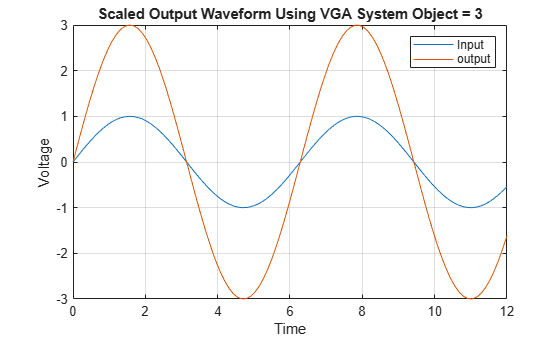# serdes.VGA

Models a variable gain amplifier

Since R2019a

## Description

The `serdes.VGA` system object scales the amplitude of the input waveform based on a gain specified by the user.

To scale the input signal:

1. Create the `serdes.VGA` object and set its properties.

2. Call the object with arguments, as if it were a function.

## Creation

### Syntax

``vga = serdes.VGA``
``vga = serdes.VGA(Name,Value)``

### Description

````vga = serdes.VGA` returns a VGA object that modifies a input waveform according to the gain defined by the user.```
````vga = serdes.VGA(Name,Value)` sets properties using one or more name-value pairs. Enclose each property name in quotes. Unspecified properties have default values.Example: `vga = serdes.VGA('Gain',5)` returns a VGA object with a multiplicative gain of 5.```

## Properties

expand all

Unless otherwise indicated, properties are nontunable, which means you cannot change their values after calling the object. Objects lock when you call them, and the `release` function unlocks them.

If a property is tunable, you can change its value at any time.

### Main

VGA operating mode, specified as `0` or `1`. `Mode` determines if the VGA adjusts the gain of input signal or acts as a pass-through.

Mode ValueVGA ModeVGA Operation
`0`Off`serdes.VGA` is bypassed, the input waveform remains unchanged.
`1`On`serdes.VGA` scales the input waveform according to the specified `Gain`.

Data Types: `double`

Multiplicative gain used to scale the input waveform, specified as a unitless scalar.

Data Types: `double`

## Usage

### Syntax

``y = vga(x)``

### Description

``y = vga(x)``

### Input Arguments

expand all

Input signal to be scaled, specified as a scalar or vector.

### Output Arguments

expand all

Scaled output signal, returned as a scalar or vector corresponding to the input signal.

## Object Functions

To use an object function, specify the System object™ as the first input argument. For example, to release system resources of a System object named `obj`, use this syntax:

`release(obj)`

expand all

 `step` Run System object algorithm `release` Release resources and allow changes to System object property values and input characteristics `reset` Reset internal states of System object

## Examples

collapse all

This example shows how to apply variable gain to input waveform using `serdes.VGA` system object™.

Create the input waveform.

```t = linspace(0,12,101); y1 = sin(t);```

Create the VGA object with a scale factor of 3.

`vga = serdes.VGA('Gain',3);`

Process the input waveform with the VGA object.

`y2 = vga(y1);`

Plot the input and output waveforms.

```figure plot(t,y1,t,y2) xlabel('Time') ylabel('Voltage') legend('Input','output') grid on title(sprintf('Scaled Output Waveform Using VGA System Object = %g',vga.Gain)) ```## Version History

Introduced in R2019a